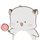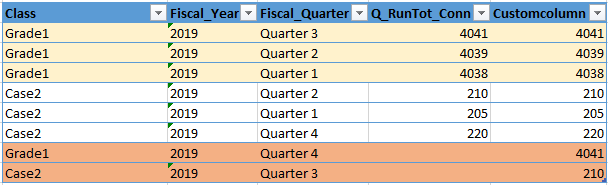cancel
Showing results for
Did you mean:Helper I

## Create new quarterly record with previous quarter data with conditions

Hello guys,

I have a data table like below. When I apply a filter (Grade1) for "Class", for the year 2019, it only has 3 quarters (q1, q2, q3). I want to create a record for q4 with the value of q3 (4041).Also for the Case2, I want to create a record for q3 with the value of q2 (210). Can I achieve this with custom columns?

1 ACCEPTED SOLUTIONCommunity Support

Hello

Please try this in the Query Editor:

``````let
Source = Table.FromRows(Json.Document(Binary.Decompress(Binary.FromText("i45Wci9KTEk1VNJRMjIwtARSgaWJRSWpRQrGQLaJgYmhUqwObkVGYEXGlngVGUIUWYAVOScWpxphN8jI0ACfEojJpviUmICEjICmxAIA", BinaryEncoding.Base64), Compression.Deflate)), let _t = ((type text) meta [Serialized.Text = true]) in type table [Class = _t, Fiscal_Year = _t, Fiscal_Quarter = _t, Q_RunTot_Conn = _t]),
#"Changed Type" = Table.TransformColumnTypes(Source,{{"Class", type text}, {"Fiscal_Year", Int64.Type}, {"Fiscal_Quarter", type text}, {"Q_RunTot_Conn", Int64.Type}}),
#"Grouped Rows" = Table.Group(#"Changed Type", {"Class", "Fiscal_Year"}, {{"Data", each _, type table [Class=text, Fiscal_Year=number, Fiscal_Quarter=text, Q_RunTot_Conn=number]}}),
in

if [Quarter]<>"4" then
List.Select({
Int64.From([Quarter]),
let temp  = Int64.From([Quarter])+1
in
if Table.RowCount(Table.SelectRows(t,each Int64.From([Quarter]) = temp))=0
then Int64.From([Quarter])+1
else null
},each _<>null) else {Int64.From([Quarter])}
), "LastQ"),"New Quarter",each "Quarter "&Text.From([LastQ]))),
#"Expanded Custom" = Table.ExpandTableColumn(#"Removed Columns", "Custom", {"Q_RunTot_Conn", "New Quarter"}, {"Q_RunTot_Conn", "Fiscal_Quarter"})
in
#"Expanded Custom"``````

The output shows:Here is my test pbix file:

I hope this helps.

Best regards

Giotto Zhi

6 REPLIES 6Community Support

Hello

Please try this in the Query Editor:

``````let
Source = Table.FromRows(Json.Document(Binary.Decompress(Binary.FromText("i45Wci9KTEk1VNJRMjIwtARSgaWJRSWpRQrGQLaJgYmhUqwObkVGYEXGlngVGUIUWYAVOScWpxphN8jI0ACfEojJpviUmICEjICmxAIA", BinaryEncoding.Base64), Compression.Deflate)), let _t = ((type text) meta [Serialized.Text = true]) in type table [Class = _t, Fiscal_Year = _t, Fiscal_Quarter = _t, Q_RunTot_Conn = _t]),
#"Changed Type" = Table.TransformColumnTypes(Source,{{"Class", type text}, {"Fiscal_Year", Int64.Type}, {"Fiscal_Quarter", type text}, {"Q_RunTot_Conn", Int64.Type}}),
#"Grouped Rows" = Table.Group(#"Changed Type", {"Class", "Fiscal_Year"}, {{"Data", each _, type table [Class=text, Fiscal_Year=number, Fiscal_Quarter=text, Q_RunTot_Conn=number]}}),
in

if [Quarter]<>"4" then
List.Select({
Int64.From([Quarter]),
let temp  = Int64.From([Quarter])+1
in
if Table.RowCount(Table.SelectRows(t,each Int64.From([Quarter]) = temp))=0
then Int64.From([Quarter])+1
else null
},each _<>null) else {Int64.From([Quarter])}
), "LastQ"),"New Quarter",each "Quarter "&Text.From([LastQ]))),
#"Expanded Custom" = Table.ExpandTableColumn(#"Removed Columns", "Custom", {"Q_RunTot_Conn", "New Quarter"}, {"Q_RunTot_Conn", "Fiscal_Quarter"})
in
#"Expanded Custom"``````

The output shows:Here is my test pbix file:

I hope this helps.

Best regards

Giotto ZhiHelper I

Thanks @v-gizhi-msft  it is working. One concern I noticed it will generate only one quarter. For example, if we have only "quarter 1" data, how to generate all four quarter based on the first quarter?Super User

In general you cannot "invent" columns using DAX. The exception is when you create an entirely new table using DAX. First question, are you trying to do this in Power Query or DAX? Second, data as text. Please see this post regarding How to Get Your Question Answered Quickly: https://community.powerbi.com/t5/Community-Blog/How-to-Get-Your-Question-Answered-Quickly/ba-p/38490. You could almost certainly do this creating a new table but you cannot invent a row using just custom columns.

Become an expert!: Enterprise DNA
External Tools: MSHGQM
Latest book!:
Mastering Power BI 2nd EditionDAX is easy, CALCULATE makes DAX hard...Helper I

@Greg_Deckler Thanks for the reply. Wouldn't mind if we can get above output using Power Query.Super User

Do you have date in this table? Then you could have done easily, with time intelligence and Date calendar

YTD and YTD till last qtr and there diff can give you this

``````YTD = CALCULATE(SUM(Table[Q run tot Count]),DATESYTD(('Date'[Date]),"12/31"))

Last QTD YTD = CALCULATE(SUM(Table[Q run tot Count]),DATESYTD(dateadd('Date'[Date],-1,Quarter),"12/31"))

This Qtr = [YTD] -[Last QTD YTD ]``````

To get the best of the time intelligence function. Make sure you have a date calendar and it has been marked as the date in model view. Also, join it with the date column of your fact/s. Refer :
https://www.archerpoint.com/blog/Posts/creating-date-table-power-bi
https://www.sqlbi.com/articles/creating-a-simple-date-table-in-dax/Helper I

@amitchandak , Thanks for the reply. I would like to create a Custom column like below,